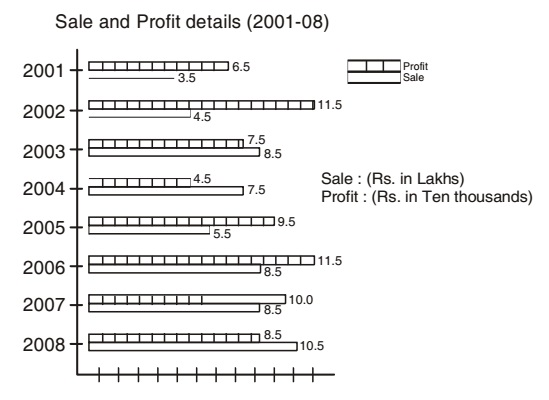Home » Data Interpretation » Bar graph » Question

#### Data Interpretation

Direction: The bar graph as shows below gives information about the sale and profit details of a departmental store during the years from 2001 - 2008. Study the graph carefully and answer the questions asked here under.1. Annual mean profit of the store (rupees in ten thousand) is approximately:
1. 8.5
2. 8.6
3. 8.7
4. 9.0
##### Correct Option: C

Annual mean profit of the store (rupees in ten thousands)
= (6.5 + 11.5 + 7.5 + 4.5 + 9.5 + 11.5 + 10.0 + 8.5)/8 = 69.5/8 =8.7 (approx.)